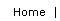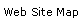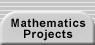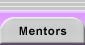# UNDERSTANDING AND CREATING DEFINITIONS

## GOALS FOR THESE ACTIVITIES:

• To discuss the structure and purpose of definitions
• To teach the skills needed to interpret definitions
• To provide practice writing definitions

Definitions play an essential role in mathematics. New mathematical objects are created by new definitions (e.g., "Let i be a number such that i2 = –1" is the start of imaginary and complex numbers). The properties with which we characterize, and distinguish between, those objects are also created by definitions (e.g., whether or not a function is periodic). Lastly, definitions help us to describe the relationship between objects (e.g., is one line perpendicular to another?). A new definition can be the start of a lengthy investigation of new mathematical questions and discoveries. For example, perfect  numbers, whole numbers whose factors sum to twice the number in question (such as 6 and 28), have been the source of many interesting investigations in number theory. However, they had to be identified and defined before they could be explored.

## UNDERSTANDING A DEFINITION

Students need to learn how to read mathematical literature (see Reading Technical Literature in the Getting Information section). Because definitions are brief, yet rich in content, they are a good place to start teaching the habits that make for successful understanding of dense mathematical writing.

Provide the following definition to the class:

A figure is convex if, for every pair of points within the figure, the segment connecting the two points lies entirely within the figure.

Note that students who informally understand the word "convex" may not be familiar with this definition or know immediately how to connect their understanding to the definition. If your class is familiar with this definition, use one of the two definitions below (be sure to reintroduce this definition of "convex" prior to the second activity below):

• A set is closed under an operation if applying that operation to members of the set always results in a member of the set.
• A set is dense if between any two distinct members of the set, there exists a third distinct element from the set. (Note that we can only talk of elements being "between" one another if the set is ordered.)

Present the students with the definition you chose, and give them a few minutes to understand it. Let them know that you will ask them for a description of the steps they took in developing their grasp of the idea. If a student just reads the sentence and stops, you can go through the questions from the Getting Stuck and Unstuck section: Do you understand the defined word? What would you need to know in order to work toward an understanding? Can you come up with a visual, numeric, or symbolic representation of any part of it?

Ask the class to describe the steps they took in studying the definition. Record their responses and organize them on the board. The following list (or a similar version) should be available to students by the end of the discussion:

1. Read the definition more than once.
2. Identify what "things" the definition is talking about. Students may note that the definition of "convex" refers to a shape and pairs of points within it. (You may want to distinguish at some point between sets of boundary points, such as circles, which are not convex, and boundaries plus their interiors, such as discs, which are convex. When we call shapes, such as regular polygons, convex, we mean the boundary plus its interior. The traditional definition of "convex" refers more abstractly to sets rather than to shapes.)
3. Generate a test case. In the definition of "convex", the words "figure" and "points" were really placeholders, so the students need to pick an example of each and then reread the definition substituting their choices. For example: A square is convex if, for every pair of points within the square, the segment connecting the two points lies entirely within the square. For a geometry definition, they should draw a diagram that matches the defined situation. In other situations, they may need to construct an example set, function, sequence, or number that might meet the defined conditions.
4. Determine whether the example fits the definition. When two specific points are chosen in the square, does their connecting segment lie within the square? This step requires consideration of different possible placement of points, such as on the boundary, close to the center, or at a vertex. It is important to note that the definition requires all cases to work and that there is an infinite number of possible pairs. Clearly, a claim that a figure is convex must be based on a sound reason rather than a few experiments. Repeat this process for several more test cases.
5. Find examples that do not fit the definition. The identification of non-examples strengthens one’s understanding of a definition and highlights the importance of each condition of the definition. Non-examples can be systematically constructed by removing each condition (or key word) of the definition in turn and finding an example that satisfies all of the remaining conditions but not the excluded one. For example, the integers are not dense, but if the condition in the definition of "dense" that the third element also be from the set is dropped, there is always a distinct real number between pairs of integers. When considering whether the reals are closed under division, a single counterexample, such as 8 ÷ 0 suffices to answer the question and emphasizes the role that "always" plays in the definition of "closed."
6. Try to generalize the examples to create an image of the full concept, and to restate or explain the definition in your own words.

As the above outline demonstrates, reading mathematics is an active process. It should be done with pen and paper available and be punctuated by the reader’s attempts to test, apply, and develop her understanding of an idea.

The next activity provides practice in reading, interpreting, and comparing definitions. After the above discussion, your class should have an informal sense that a figure is convex if it has no dents; for example,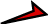is non-convex. Provide the list of possible definitions below and ask which of them would also effectively distinguish convex from non-convex polygons. You may want to have the class study a few of the definitions in pairs and then try a few individually. After students have worked on the Definition A, have them share their test cases with the class. Do the examples lead them to accept A as an alternative definition? Can they justify a claim that the rule must apply for all convex polygons and fail for all non-convex ones? A careful reading and testing of all seven possible definitions should take quite a while, so you might assign what students don’t finish in class as homework.

 Possible Definitions for "Convex" A polygon is convex if and only if… All diagonals lie in the interior of the polygon. The perimeter is larger than the length of the longest diagonal. Every diagonal is longer than every side. The perimeter of the polygon is the shortest path that encloses the entire shape. The largest interior angle is adjacent to the longest side. None of the lines that contain the sides of the polygon pass through its interior. Every interior angle is less than 180°.

If, during the follow-up discussion, students disagree about whether a definition is satisfactory, ask for their evidence. A definition should be rejected if a convex shape can be given that fails to meet the stated conditions. Because of the bi-directional nature of a definition, a rule should also be rejected if a non-convex example can be provided that does satisfy the stated conditions. For example, this figure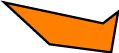demonstrates that Definition E does not describe only convex shapes. Both of the figures below disqualify Definition C. Ask the class to explain why.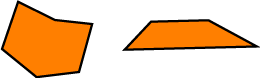Students’ tendencies to only look for confirming examples can be revealed by their work on Definition B. This condition describes any convex shape they may test; however, it is also true for all non-convex shapes as well (due to the triangle inequality) and, therefore, lacks the necessary specificity of a good definition.

## EVALUATING DEFINITIONS

Definitions A, D, F, and G all satisfactorily distinguish convex polygons from their non-convex brethren. Which version of a definition mathematicians choose to use is influenced by several considerations. The class should discuss the following questions:

• Which of the four definitions that work is best? Why? (Students may note that some are clearer, shorter, or easier to test. They may also consider them easier to visualize than the original definition.)
• Why don’t we use one of these as the standard definition instead? (Draw a curvilinear shape [one that lacks distinct vertices, diagonals, and sides] and have students try to test it for convexity, using the definitions. Have them try to rewrite at least two of the four so that they work with all closed planar figures [you can ask them to generate a list of convex and non-convex test cases ahead of time and put them on the board]. Have students proofread one another’s efforts. This sequence will make it clear that only certain definitions can be generalized to all two-dimensional figures.)
• Does the idea of convexity apply in three dimensions? Can students write a definition for convex polyhedra (shapes with polygonal faces, such as cubes or pyramids)? For any three-dimensional region? (Point out that the original definition works well in all dimensions. Even though it may be more difficult to first interpret, it was chosen because of its generality.

## WRITING DEFINITIONS

Felix: Love is that intense emotional feeling that one person has for another. Oscar: I feel that way about you and I’m pretty sure it’s hate.

- The Odd Couple (television show)

The above activities highlight the value of clearly written and tested definitions. It is instructive for students to attempt writing their own definitions of familiar objects or concepts. Once they understand that creating any definition can be challenging, they are more likely to appreciate the effort required in creating good mathematical definitions.

Almost any word can be posed as a definitional challenge. "Love," "democracy," or "good" are predictably fun when the class attempts to come to a consensus. But even seemingly mundane objects can be problematic. Choose a word you like or hand out the lyrics to Paul Simon’s circularly defined song Cars Are Cars (or, even better, provide the lyrics and play the song for the class). In the latter case, you can note that using a word to describe itself, as the title does, fails to provide any useful information. Ask your students to each write a clear definition of "car," and then have them write their definitions on the board. Solicit general or specific comments. For example, students may note the range of approaches, the different degrees of detail, or a particular criterion in someone’s definition.

 Cars Are Cars Cars are cars All over the world Cars are cars Similarly made Similarly sold In a motorcade Abandoned when they're old Cars are cars All over the world Cars are cars All over the world Engine in the front Jack in the back Wheels take the brunt Pinion and a rack Cars are cars All over the world But people are strangers They change with the curve From time zone to time zone As we can observe They shut down their borders And they think they're immune They stand on their differences And shoot at the moon But cars are cars All over the world Drive 'em on the left Drive 'em on the right Susceptible to theft In the middle of the night Cars are cars All over the world Cars are cars All over the world I once had a car That was more like a home I lived in it, loved in it Polished its chrome If some of my homes Had been more like my car I probably wouldn't have Traveled this far Cars are cars All over the world Cars are cars All over the world Copyright © 1983 by Paul Simon. From the album Hearts and Bones.

Ask the class to use the definitions on the board to discuss what we mean when we say a definition should be clear. Answers should include that it is not overly long. A too-detailed definition will exclude cases that should be acceptable. For example, in one discussion, students objected to the requirements that cars have four wheels (they had seen one with three), have a steering wheel (a joystick could work), or be powered by gasoline. The criteria that emerge can be described as follows: Definitions should:

• avoid unnecessary words or conditions; unwieldy definitions become unclear and overly limiting.
• unambiguously define the thing of interest (i.e., say enough to exclude non-examples of the concept).
• only use words whose meanings themselves are clear or accepted as understood.

This trio of conditions can be remembered as the Goldilocks rules (i.e., you don’t want a definition to be too big or too little, but just right). The last of these conditions requires some explanation. In mathematics, it becomes the requirement that we only use words that are previously defined or intentionally left undefined. We must have some undefined words in order to avoid an infinite process of definitions or a circular chain of definitions. (Common undefined words in mathematics are "set," "point," and "line.")

Students can discover the need for undefined terms themselves. Have them pick a word they would like to define, define it, and then define each key word that they used in their definitions. Have them then define any key words from the second round of definitions and repeat that process indefinitely. Sooner or later, they will quit or re-use either the original or one of the subsequently defined words. If they believe this predicament is due to a personal failing, show them how quickly even dictionaries lapse into circularity (see the box below). At the end of this activity, it is fun for the class to consult dictionaries for definitions of "car" (as well as "automobile," which will probably be closer to the class definition).

 A Dictionary Attempt to Define "Dimension" Dimension: Any measurable extent, such as length, width, depth. Extent: The space, amount, or degree to which a thing extends; size; length; breadth. Measurement: Extent, quantity, or size as determined by measuring. Size: That quality of a thing that determines how much space it occupies; dimensions; extent. Length: The measure of how long a thing is; the greatest of the two or three dimensions of anything; extent in space. . . . and so on! - From Webster’s New World Dictionary, Second College Edition

The Goldilocks criteria are necessary conditions for a good definition, but they are not always sufficient. A good definition must also describe an object or property that has some interest. A definition might accurately define an object that does not exist (e.g., tetratris are triangles with four axes of symmetry)! A definition might also be valid but less useful than possible. For example, students often ask why 1 is not considered a prime number. It certainly has no other factors besides 1 and itself. However, the choice is based on the clarity that arises from 1’s exclusion. Many theorems about primes would need to list 1 as an exception, were it counted among the primes. For instance, the fundamental theorem of arithmetic states that every positive integer can be uniquely decomposed into prime factors. The uniqueness of the set, which is a powerful result, would be sacrificed if 1 were prime (e.g., 6 would equal both 2 x 3 as well as 1 x 2 x 3). The inclusion or exclusion of degenerate cases (those lacking the full messiness of the main idea) is often an important step in crafting a valuable definition.

The degenerate case decision arises regularly. Should squares be considered rectangles, or should rectangles be defined as having adjacent sides of unequal length? It is better to allow inclusion because any theorem proven for rectangles will automatically apply to squares. Such hierarchical definitions are motivated by a "two (or more) for the price of one" objective. Each decision is made, often after the fact, once more is known about the concept. "Carl’s Question," below, arose during a discussion of degenerate cases. Once students discover that only Theorems C and F remain valid, given Carl’s new definition of a triangle, they are likely to agree that it is more efficient to consider flat triangles separately.

 Carl’s Question Carl wanted to know why the definition of a triangle should not allow triangles to be flat. He thought that three collinear points and their connecting segments (see "Triangle ABC," below) should be acceptable because this figure satisfied the angle sum theorem (there are two 0° angles and one 180° angle).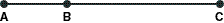"Triangle" ABC Which of the following theorems are not true for all triangles if flat triangles are allowed? The perpendicular bisectors of the sides of a triangle all intersect at a single point (are coincident). If two sides of a triangle are unequal, then the angles opposite them are unequal. The exterior angle at one vertex is equal in measure to the sum of the other two angles of the triangle. Two triangles are congruent if two angles and the included side of one triangle are congruent to two angles and the included side of another triangle. The sum of the lengths of any two sides of a triangle is greater than the length of the third side. If two angles of a triangle are unequal, then the sides opposite them are unequal.

Once the class has tackled defining everyday words, they should practice writing technical mathematics definitions. Any of the four examples below can be used (omit the "Possible Conditions" column, which is for your own use). Each problem provides objects that are, and are not, members of a set. Students should look for properties that distinguish between the two groups and try to write a single definition for the objects in the left-hand box (e.g., A figure is an Omino if it consists of congruent squares…). Classmates should read one another’s definitions and check that they apply to all of the figures on the left and none on the right. Note that this activity requires an inductive assumption. Your students will be trying to create, from a small number of examples, some larger rule. Their definitions may work for these sets and still differ from one another. There is no unique interpretation (although we may recognize familiar concepts illustrated).

For each definition, have students identify which of the words used are already defined (e.g., known from basic geometry studies), are simple English words ("the," "if," etc.), need to be defined, or should be made officially undefined (ideally, as few as possible). Encourage them to be as picky with one another as possible. Offer your own attempts.

Many student definitions will involve words that themselves require defining. For example, what does it mean for squares to "share" an edge? How do you know a region is "inside" a curve? What is a "loop?" Ask everyone to write a definition for one word they used that lacks a technical definition. These efforts will help emphasize that definitions are frequently based on earlier definitions (just as theorems are built on prior results).

Often, a definition is more readily understood when we substitute the definition of a previously defined term for the term itself, or at least review the supporting definition. For example, the definition of "dense" (in the Understanding a Definition section) uses the word "between." Sometimes students assume that "between" means "at the midpoint." Looking up the definition of "between" (rather than applying an everyday interpretation) ensures that students were sticking with the mathematical sense of the word.

 Ominoes Not Ominoes Possible Conditions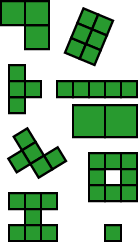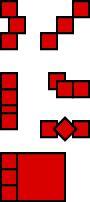A single square or a set of congruent squares. All share a common edge with at least one other square. Shared edges overlap completely (from vertex to vertex). No overlap of interiors.

 Trees Not Trees Possible Conditions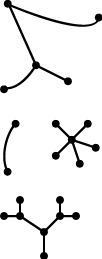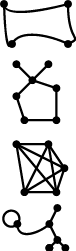A set of segments and arcs (with big endponts?!). No closed regions. No loops or cycles. No common points except at the big endpoints. Fewer arcs and segments than big endpoints.

 Items Not Items Possible Conditions –17 x2 – 323x + 12.3 b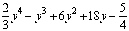9 + 2x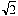t8 – 1 5x 87 + x52 – x24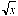2m2.5 + 7m – 3 4y2 –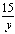d0.2 3x2 + logx 12x Only whole number exponents (so no variables in roots or denominators). The sum (and difference) of terms that are a constant times a variable to a whole power.

 Things Not Things Possible Conditions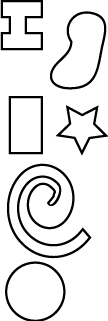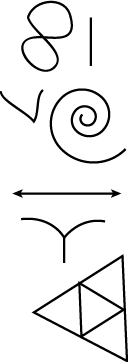A closed shape. Exactly one inside (can they define what makes a region inside or outside?). No intersection (how defined?). No endpoints. A single path (how defined?).

## CREATING NEW DEFINITIONS TO CREATE NEW PROBLEMS

New definitions are an invitation to new problems. Students looking for new questions to pursue should try defining new objects, properties, or relationships. Give your class the definitions of "semicenter" and "semicentral":

A point is a semicenter of a set if the segments connecting the point to every other point of the set lie entirely within the set. A figure with a semicenter is semicentral.

You can ask them to draw figures that are and are not semicentral. For semicentral shapes, have them identify (e.g., color in) their semicenters. If necessary, you can then use the figures below as additional test cases (B and D are not semicentral).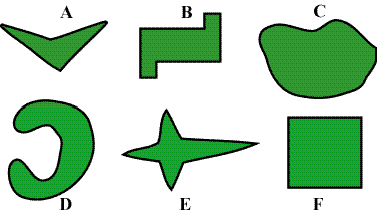Some questions may arise in the course of seeking examples and coloring the set of semicenters. Ask sudents to write down as many new questions as they can about such figures. Here are some possibilities:

• How do you find all of the semicenters?
• What, if anything, do non-semicentral shapes have in common?
• What, if anything, do semicentral shapes have in common?
• Can a figure have a single semicenter? If so, can such a uni-semicentral figure have its semicenter on its boundary? In its interior?
• What is the fewest number of sides a unisemicentral polygon could have?
• Can a figure have a finite number of semicenters greater than one?
• What if all of the points in a figure are also semicenters? (Called toti-semicentral? No, just convex!)
• Is the set of semicenters always connected? Convex?
• Can the semicenter set be a triangle? A square? A circle? What shapes can be a semicenter set (as a proper subset of the shape)? Is there a unique starting figure for some such sets?
• Can a region with a hole have a semicenter?
• What questions arise regarding n-dimensional semicentral shapes?

Do not expect an extensive list on the first try. If only a few questions arise at first, provide the class with some time to explore those questions. As they seek answers and further study the shapes, they are likely to pose new questions in response to their investigations. Have them share their questions and conjectures with one another as they arise, and, for settings that capture a class’s imagination, the process will snowball.

Definitions can arise in different ways. "Semicentral" was created because of a desire for a new definition to play with, rather than being motivated by ongoing research. Definitions that are created to describe an important or complex idea that is emerging from a lengthy effort are, in general, more likely to lead to deeper discoveries and more varied connections. Nonetheless, students can open many avenues of research simply by tweaking known definitions or inventing new arithmetic or geometric properties out of whole cloth.

The act of inventing a definition requires creativity and precision. Students should be encouraged to be playful. As they venture into the formal work of proving their claims, new definitions will enable them to make proofs that are clearer, simpler, and more convincing.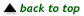Translations of mathematical formulas for web display were created by tex4ht. © Copyright 2003 Education Development Center, Inc. (EDC)